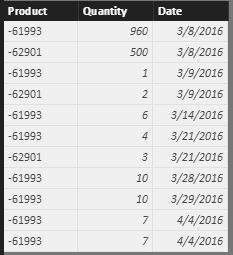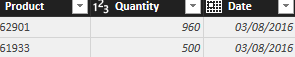cancel
Showing results for
Did you mean:Helper I

## Can't show values under all Date Columns?! Help Please

I am trying to create a running stock total for a number of products over time. I am using two data values (qty), the first is associated with a date (Incoming deliveries), the second is a current stock level and is therefore not associated with a date.

After building a matrix to display products in the rows and date/weeks in columns, I have attempted to create a calculated measure to show the running total combining current stock level and incoming deliveries. The measure is a simple calculate expression, and the outcome is correct, however, the output values only appear in my matrix under the week columns where there are incoming deliveries.

Is there a way that I can show the stock level under all weeks, and not just those with incoming deliveries?

1 ACCEPTED SOLUTIONMicrosoft

@J_R_Cribb

If there are no incoming deliveries, the product data will not exist for that week in your dataset, right?

In following test dataset, there is no incoming deliveries for “-62901” on 3/14, 3/28, 3/29 and 4/4.We can first create a new table with following formula. Relate this new table with stock table using product.

```FullTable =
GENERATE (
SUMMARIZECOLUMNS ( Table2[Product] ),
SUMMARIZECOLUMNS ( Table2[Date] )
)```

Then create WeekNum column and Quantity column in this new table.

```WeekNum =
WEEKNUM ( FullTable[Date] )```
```Quantity =
CALCULATE (
SUM ( Table2[Quantity] ),
FILTER (
Table2,
Table2[Date] = FullTable[Date]
&& Table2[Product] = FullTable[Product]
)
)```

At last, create a measure with following formula. I’ve also upload my .pbix file here for reference.

```LastNoBlankTotal =
VAR LastNoBlankWeek =
CALCULATE (
MAX ( FullTable[WeekNum] ),
FILTER (
ALL ( FullTable ),
FullTable[WeekNum] < MAX ( FullTable[WeekNum] )
&& FullTable[Quantity] <> BLANK ()
),
VALUES ( Table1[stp_product] )
)
RETURN
(
IF (
[Incoming Qty] = BLANK (),
[Stock Qty]
+ CALCULATE ( [Incoming Qty], FullTable[WeekNum] = LastNoBlankWeek ),
[Rolling Total]
)
)```Best Regards,

Herbert

7 REPLIES 7Microsoft

@J_R_Cribb

Could you please provide a simple data sample and the calculated measure you created to us? So that we can know more clearly about you problem.

Best Regards,

HerbertHelper I

@v-haibl-msft

Thank you for your response. I have the two sets below (simplified).I have created a calculated column to withdraw the week number from the bottom table (incoming), and there is a relationship between the incoming table and stock table.

I am laying out as below:As you can see, I have my product as rows, week number as columns. I have created measures for the Incoming Qty and Stock Qty, and the simple calculation I have used is: Rolling Total = [Stock Qty] + [Incoming Qty].

I'd like to see the blank weeks filled in, but I can't seem to achieve it having tried various calculations and seeking other advice on the forum.

Thank you!Microsoft

@J_R_Cribb

So you want to fill the blank with value of previous week? For example, the value of ‘product -61993’ and ‘week number 36’ should be -30?

BTW, what is the formula of your measures for the Incoming Qty and Stock Qty?

Best Regards,

HerbertHelper I

@v-haibl-msft

Yes that's exactly what I would like to do!

My measures are just a SUM of the original value - I don't know whether this was necessary but in my mind made them easier to work with.

Thank you Herbert,

JMicrosoft

@J_R_Cribb

If there are no incoming deliveries, the product data will not exist for that week in your dataset, right?

In following test dataset, there is no incoming deliveries for “-62901” on 3/14, 3/28, 3/29 and 4/4.We can first create a new table with following formula. Relate this new table with stock table using product.

```FullTable =
GENERATE (
SUMMARIZECOLUMNS ( Table2[Product] ),
SUMMARIZECOLUMNS ( Table2[Date] )
)```

Then create WeekNum column and Quantity column in this new table.

```WeekNum =
WEEKNUM ( FullTable[Date] )```
```Quantity =
CALCULATE (
SUM ( Table2[Quantity] ),
FILTER (
Table2,
Table2[Date] = FullTable[Date]
&& Table2[Product] = FullTable[Product]
)
)```

At last, create a measure with following formula. I’ve also upload my .pbix file here for reference.

```LastNoBlankTotal =
VAR LastNoBlankWeek =
CALCULATE (
MAX ( FullTable[WeekNum] ),
FILTER (
ALL ( FullTable ),
FullTable[WeekNum] < MAX ( FullTable[WeekNum] )
&& FullTable[Quantity] <> BLANK ()
),
VALUES ( Table1[stp_product] )
)
RETURN
(
IF (
[Incoming Qty] = BLANK (),
[Stock Qty]
+ CALCULATE ( [Incoming Qty], FullTable[WeekNum] = LastNoBlankWeek ),
[Rolling Total]
)
)```Best Regards,

HerbertHelper I

@v-haibl-msft

Please could you provide an explanation on how your formulae for LastNoBlankWeek & LastNoBlankTotal operate?

Thank you,

JHelper I

@v-haibl-msft

Hi Herbert,

Thank you for detailing this perfectly - I've tried and tested for all products that I have data for (100+) and it gives me a running total which I couldn't achieve before.

If I filter from a certain date, say Week 33 for instance, would it only take into account future data points?

I also have a third table giving descriptions for each product code, which is linked to both data tables. Whenever I have tried to include the description in the table it either crashes or just does not work - is this perhaps a memory issue due to the vast number of calculations that BI needs to do?

Thank you again,

J